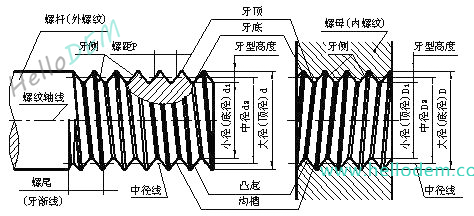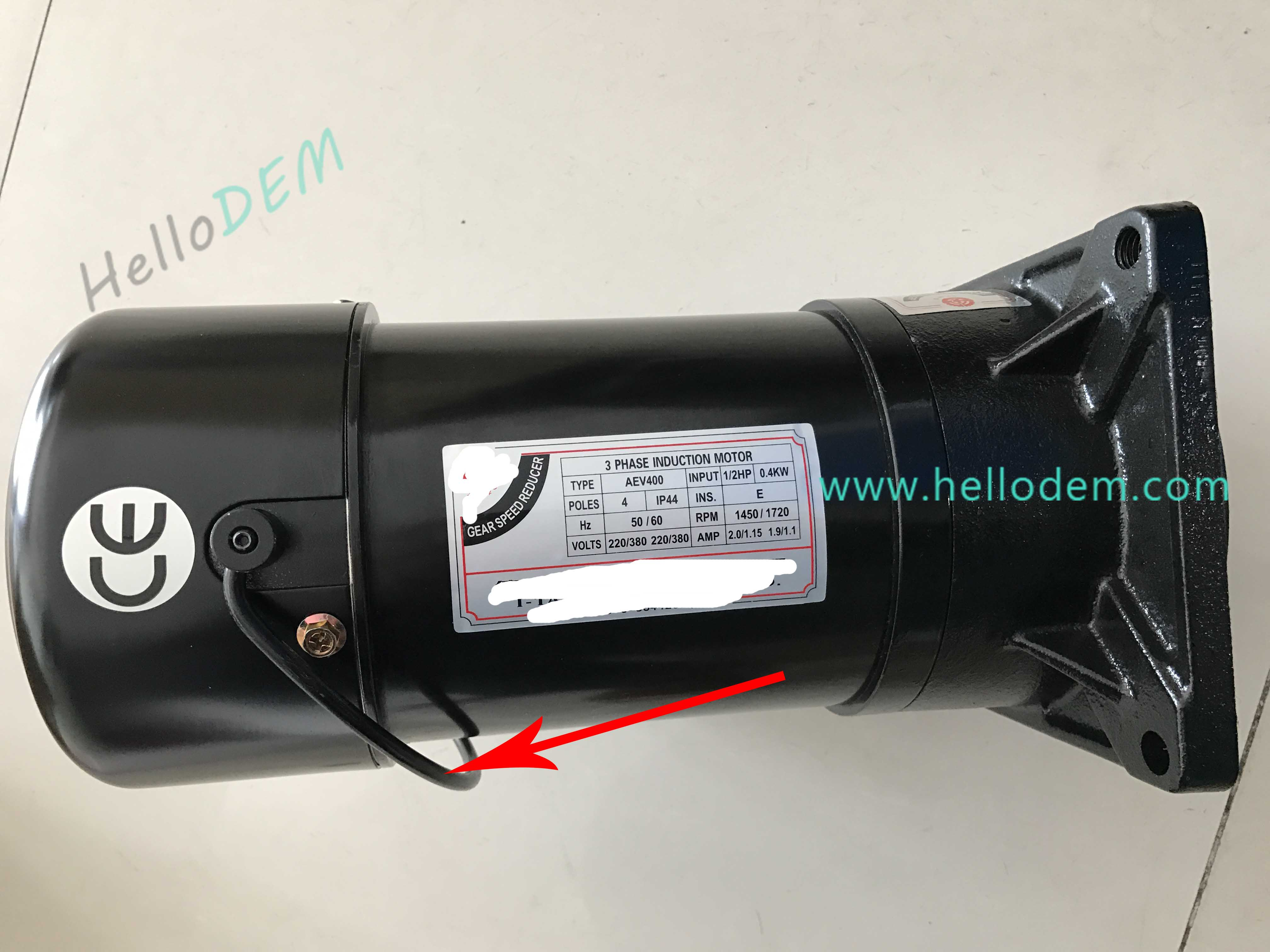G92 X（U）__ Z（W）__ R__F__；

X、Z：螺纹终点的绝对坐标值（U、W表示增量值）。

R：为锥螺纹大端和小端的半径差。

F：导程（单线螺纹的螺距等于导程）。

G84（右旋）X_Y_Z_R_P_F_K/L_

X_Y_：孔中心坐标

Z底孔坐标

R参考平面高度

P暂停时间(单位:毫秒)

F切削进给速度

K/L重复次数（相对坐标有效）

K（锥度）=2tga（=2 x tg斜角)=D - d / l（大头直径 - 小头直径 / 锥体长度）。

D（大头直径）=b + 2ltga（=小头直径 + 2 x 锥体长度 x tg钭角）=d + Kl（=小头直径 + 锥度 x 锥体长度）=d + 2lM（=小头直径 + 2 x 锥体长度 x 斜度）

d（小头直径）=D-2ltga（=大头直径-2x锥体长度xtg钭角）=D - Kl（=大头直径 - 锥度 x 锥体长度）=D - 2lM(=大头直径 - 2 x 锥体长度 x 斜度）。##### 工程训练加工中心实训报告练习题目##### 用G66调用宏程序加工孔的数控编程实例##### 加工中心刀库换刀时，机械臂卡刀的处理方法（视频教程）